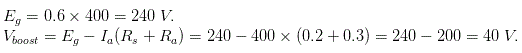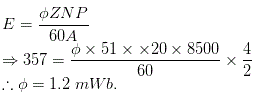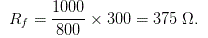# MCQs on Electrical Machines

##### Page 51 of 114. Go to page 1 2 3 4 5 6 7 8 9 10 11 12 13 14 15 16 17 18 19 20 21 22 23 24 25 26 27 28 29 30 31 32 33 34 35 36 37 38 39 40 41 42 43 44 45 46 47 48 49 50 51 52 53 54 55 56 57 58 59 60 61 62 63 64 65 66 67 68 69 70 71 72 73 74 75 76 77 78 79 80 81 82 83 84 85 86 87 88 89 90 91 92 93 94 95 96 97 98 99 100 101 102 103 104 105 106 107 108 109 110 111 112 113 114
01․ A series generator is connected in series to a feeder having 0.5Ω resistance to boost the voltage. The induced voltage is given by Eg = 0.6Ia, Ra = 0.2Ω and Rs = 0.3Ω. Find the voltage boosted by the generator, when delivering 400 Amp?
30 V.
40 V.
60 V.
90 V.02․ When an electric train is moving down a hill, the DC motor will operate as
DC series motor.
DC series generator.
DC shunt motor.
DC shunt generator.

Normally in electric traction DC series motors are employed. At above condition the back emf is greater than supply voltage hence it will operate as series generator.

03․ The DC generator runs at a speed of 1000 rpm and flux per pole is 0.2 Wb, number of poles are 2 the winding is wave wound and having 480 conductors. The critical speed is 1200 rpm. Then the generated emf ( Eg )
0 Volt.
1500 Volt.
1600 Volt.
2000 Volt.

If the critical speed of machine is less than the speed of the machine, then only emf will generate. In above case critical speed is greater than speed of machine. Hence no emf will generates i.e., 0 V.

04․ A 4 pole dynamo with wave wound armature has 51 slots contains 20 conductors in each slot. If induced emf 357 Volts and speed is 8500 rpm, flux per pole will be
1.2 mWb.
1.4 mWb.
2.7 mWb.
3.5 mWb.05․ What is the full form of MNP?
Magnetic neutral pole.
Magnetic neutral plane.
Magnetic field neutral pole.
Magnetic field neutral plane.

The plane through the axis, along which no emf is induced in the armature conductor is known as MNP ( Magnetic neutral plane ).

06․ A shunt generator has a critical field resistance of 300 Ω at a speed of 800 rpm if the speed is raised to 1000 rpm the critical field resistance is
375 Ω.
225 Ω.
150 Ω.
145 Ω.

Given critical resistance = 300 Ω at 800 rpm. For generator, Eg ∝ N. So the critical field resistance also increases slightly with speed.07․ The polarity of a DC generator can be reversed by
reversing the field current.
increasing field current.
reversing field electric current as well as direction of rotation.
any of above.

In a DC generator, if we reverse the field current then the polarities of voltage generated is reversed as the current flows in opposite direction.

08․ The principle of dynamically induced emf is utilized in
transformer.
choke.
generator.
thermocouple.

In generator emf is generated due to relative motion between a magnetic field and rotation of conductors. So the emf generated is dynamically induced emf.

09․ Emf induced in a coil rotating in a uniform magnetic field will be maximum when the
flux linking with the coil is maximum.
rate of change flux linkage is maximum.
rate of change flux linkage is minimum.
rate of cutting flux by the coil sides is minimum.

According to Faraday’s law of electromagnetism, emf induced in a coil rotating in a uniform magnetic field is directly proportional to the rate of change of flux linkage. So emf induced will be maximum when rate of change of flux linkage is maximum.

10․ Magnetic field in a DC generator is produced by
electromagnets.
permanent magnets.
both of above.
none of above.

Magnetic field in DC generators is produced by passing current through the field windings present on the core of the stator. So the magnetic field is produced by electromagnets.

<<<4950515253>>>